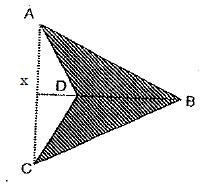This blog is managed by Song Hock Chye, author of Improve Your Thinking Skills in Maths (P1-P3 series), which is published and distributed by EPH.

## Sunday, July 18, 2010

### ACS Primary 2009 PSLE Math Prelim Paper 2 Q10

Given that the length of DB is 14 cm and the shaded region is 115 square cm, find the lenght of AC, correct to 2 decimal places.SolutionArea of Triangle = Half base x height.
Area of Triangle ABC --> (1/2)(AC)(DX + 14)
Area of Triangle ACD --> (1/2)(AC)(DX)

--> Area of Triangle ABC - Area of Triangle ACD = 115

(1/2)(AC)(DX + 14) - (1/2)(AC)(DX) = 115

(1/2)(AC)(DX) + (1/2)(AC)(14) - (1/2)(AC)(DX) = 115

(1/2)(AC)(14) = 115
7(AC) =115
AC = 115 divided by 7
= 16.428
~ 16.43 (nearest to 2 decimal places)